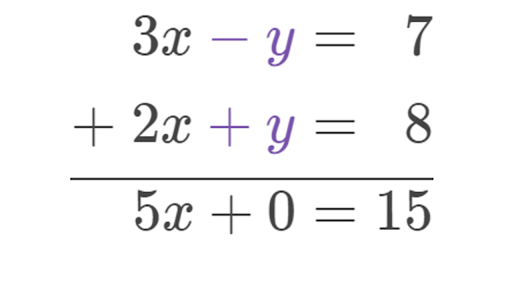# Day: May 28, 2021#### Linear Equations in One Variable with Examples

May 28, 2021

Definition of Linear Equation in One Variable: A linear equation in one variable is an equation that can be written in the form ax+b =0. Here a and b are real numbers. Linear equations also happen to be first-degree equations because the exponent on the variable is understood to be 1. Objective 1: Identify Equations […]